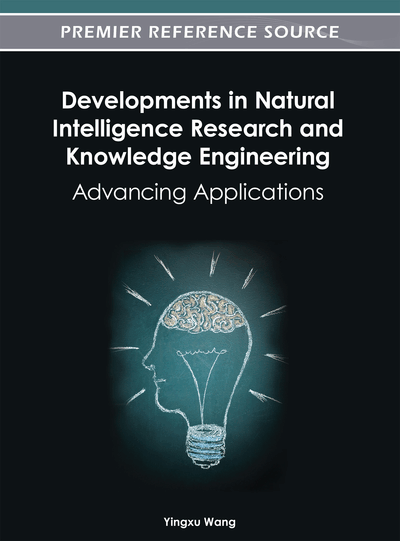# Some Remarks on the Concept of Approximations from the View of Knowledge Engineering

Tsau Young Lin (San Jose State University, USA), Rushin Barot (San Jose State University, USA) and Shusaku Tsumoto (Shimane Medical University, Japan)
DOI: 10.4018/978-1-4666-1743-8.ch020

## Abstract

The concepts of approximations in granular computing (GrC) vs. rough set theory (RS) are examined. Examples are constructed to contrast their differences in the Global GrC Model (2nd GrC Model), which, in pre-GrC term, is called partial coverings. Mathematically speaking, RS-approximations are “sub-base” based, while GrC-approximations are “base” based, where “sub-base” and “base” are two concepts in topological spaces. From the view of knowledge engineering, its meaning in RS-approximations is rather obscure, while in GrC, it is the concept of knowledge approximations.
Chapter Preview
Top

## Rs-Approximations In (Infinite) Universe

The approximation theory of RS is well known. For preciseness, we will recall the notion here. Let U be a classical set, called the universe. Letbe a partition, namely, a family of subsets, called equivalence classes, that are mutually disjoint and their union is the whole universe U. Then the pair (U,) is called approximation space in RS. Pawlak introduced following two definitions. Observe that Pawlak focus on finite universe. However we allow U to be infinite.

Let X be an arbitrary subset of the universe U.

Definition (RS) 1 Let E be an arbitrary equivalence class of R.

This definition is the formal form of the intuitive upper and lower approximations

Definition (RS)2 Let p be an arbitrary element of U.

In RS community, the previous definitions are directed generalized to Covering Cov by interpreting E as member of Cov .

Top

## Counter Intuitive Phenomena

In this section, we present some Counter Intuitive phenomena of approximations. The first example was generated to answer some questions raised in a conversation with Tian Yang, Guangming Lang, Jing Hao from Hunan University.

## Complete Chapter List

Search this Book:
Reset